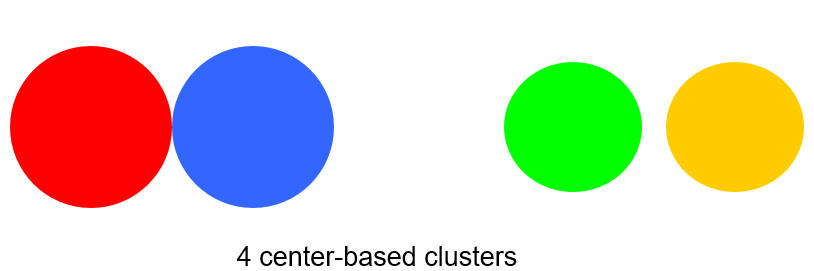top of page
Search

# Clustering Assignment Help, Project Help and Homework Help | Hire Dedicated Machine Learning Experts

If you face any issue in your machine learning clustering related projects, homework's or assignments then don't worry about it. Realcode4you is group of top rated dedicated Machine Learning experts.

Here we first learn about clustering.

What is Clustering?

How do I group these documents by topic?

How do I group my customers by purchase patterns?

Sort items into groups by similarity:

• Items in a cluster are more similar to each other than they are to items in other clusters.

• Need to detail the properties that characterize “similarity”

Not a predictive method; finds similarities, relationships

Our Example: K-means Clustering

What is Cluster Analysis?

Finding groups of objects such that the objects in a group will be similar (or related) to one another and different from (or unrelated to) the objects in other groups.Types of Clusters: Well-Separated

Well-Separated Clusters:

• A cluster is a set of points such that any point in a cluster is closer (or more similar) to every other point in the cluster than to any point not in the cluster.Types of Clusters: Center-Based

Center-based

• A cluster is a set of objects such that an object in a cluster is closer (more similar) to the “center” of a cluster, than to the center of any other cluster

• The center of a cluster is often a centroid, the average of all the points in the cluster, or a medoid, the most “representative” point of a clusterK-Means Clustering - What is it?

Used for clustering numerical data, usually a set of measurements about objects of interest.

Input: numerical. There must be a distance metric defined over the variable space.

• Euclidian distance

Output: The centers of each discovered cluster, and the assignment of each input to a cluster.

• Centroid

What Euclidian Distance?K-means Clustering

Characteristics

• Partitional clustering approach

• Each cluster is associated with a centroid (center point)

• Each point is assigned to the cluster with the closest centroid

• Number of clusters, K, must be specified

• The basic algorithm is very simple

Algorithm:K-means Clustering – Details

- Initial centroiInitial centroids are often chosen randomly.

• Clusters produced vary from one run to another.

- The centroid is (typically) the mean of the points in the cluster.

- ‘Closeness’ is measured by Euclidean distance, cosine similarity, correlation, etc.

- K-means will converge for common similarity measures mentioned above.

- Most of the convergence happens in the first few iterations.

- Often the stopping condition is changed to ‘Until relatively few points change clusters’

• Complexity is O( n * K * I * d )

n = number of points, K = number of clusters, I = number of iterations, d = number of attributesds are often chosen randomly.

Use Cases

Often an exploratory technique:

• Discover structure in the data

• Summarize the properties of each cluster

Sometimes a pre-step to classification:

• "Discovering the classes“

Examples

• The height, weight and average lifespan of animals

• Household income, yearly purchase amount in dollars, number of household members of customer households

• Patient record with measures of BMI, HBA1C, HDL

Diagnostics – Evaluating the Model

Do the clusters look separated in at least some of the plots when you do pair-wise plots of the clusters?

• Pair-wise plots can be used when there are not many variables

Do you have any clusters with few data points?

• Try decreasing the value of K

Are there splits on variables that you would expect, but don't see?

• Try increasing the value K

Do any of the centroids seem too close to each other?

• Try decreasing the value of K